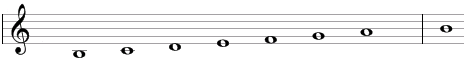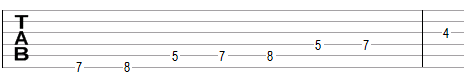# Locrian scale

The Locrian scale is a primary heptatonic scale that can be built using the following steps between notes: ½, 1, 1, ½, 1, 1, 1.

One example of this scale is B, C, D, E, F, G, A. This example is shown below in traditional notation and in guitar tablature notation.The Locrian scale is the seventh mode of the standard major scale or the second mode of the natural minor scale.

The Locrian scale is a "primary" heptatonic scale (heptonia prima) as the intervals between any two adjacent notes are either one or two semitones (1 step or ½ steps) and as the two single-semitone intervals are spaced as evenly as possible. If we start from the single-semitone interval between B and C and go up we will get the two intervals of two semitones between C and D and D and E and then get to the second interval of one semitone between E and F. If we do the same in the opposite direction, we will go through three intervals of two semitones – between A and B, G and A, and F and G.

## Modes of the Locrian scale

The following are modes of the Locrian scale.

1. Locrian, with steps ½, 1, 1, ½, 1, 1, 1 (B, C, D, E, F, G, A in the example above).
2. Ionian or major, with steps 1, 1, ½, 1, 1, 1, ½ (C, D, E, F, G, A, B).
3. Dorian, with steps 1, ½, 1, 1, 1, ½, 1 (D, E, F, G, A, B, C).
4. Phrygian, with steps ½, 1, 1, 1, ½, 1, 1 (E, F, G, A, B, C, D).
5. Lydian, with steps 1, 1, 1, ½, 1, 1, ½ (F, G, A, B, C, D, E).
6. Mixolydian, with steps 1, 1, ½, 1, 1, ½, 1 (G, A, B, C, D, E, F).
7. Aeolian or natural minor, with steps 1, ½, 1, 1, ½, 1, 1 (A, B, C, D, E, F, G).

## Three-note chords on the Locrian scale

The following are common triads built on the notes of the Locrian scale.

• On root of the scale (on the tonic): diminished chord (e.g., Bdim composed of B, D, F).
• On the second note (on the supertonic): major chord (C = C, E, G) or suspended chords (Csus4 = C, F, G and Csus2 = C, D, G).
• On the third note (on the mediant): minor chord (Dm = D, F, A) or suspended chords (Dsus4 = D, G, A and Dsus2 = D, E, A).
• On the fourth note (on the subdominant): minor chord (Em = E, G, B) or suspended chord (Esus4 = E, A, B).
• On the fifth note (on the dominant): major chord (F = F, A, C) or suspended chord (Fsus2 = F, G, C).
• On the sixth note (on the submediant): major chord (G = G, B, D) or suspended chords (Gsus4 = G, C, D and Gsus2 = G, A, D).
• On the seventh note (on the leading tone): minor chord (Am = A, C, E) or suspended chords (Asus4 = A, D, E and Asus2 = A, B, E).

## Four-note chords on the Locrian scale

The following are seventh chords built on the notes of the Locrian scale.

• On the first note: half-diminished seventh chord (minor seventh chord with a flat fifth, Bm7b5 composed of B, D, F, A).
• On the second note: major seventh chord (Cmaj7 = C, E, G, B).
• On the third note: minor seventh chord (Dmin7 = D, F, A, C).
• On the fourth note: minor seventh chord (Emin7 = E, G, B, D).
• On the fifth note: major seventh chord (Fmaj7 = F, A, C, E).
• On the sixth note: dominant seventh chord (G7 = G, B, D, F).
• On the seventh note: minor seventh chord (Amin7 = A, C, E, G).

## Intervals on the Locrian scale

The Locrian scale is composed of the following intervals.

• A minor second, e.g., the interval between B and C is equal to one semitone.
• A minor third, the interval between B and D is equal to three semitones.
• A perfect fourth, the interval between B and E is equal to five semitones.
• A diminished fifth, the interval between B and F is equal to six semitones.
• A minor sixth, the interval between B and G is equal to eight semitones.
• A minor seventh, the interval between B and A is equal to ten semitones.

Scale, Scale (index)

### Filtered HTML

• Freelinking helps you easily create HTML links. Links take the form of [[indicator:target|Title]]. By default (no indicator): Click to view a local node.